Corollary of Leibniz rule for Lie bracket

(diff) ← Older revision | Latest revision (diff) | Newer revision → (diff)

Statement

This is an identity that uses the Leibniz rule to measure the failure of the Lie bracket operation from being$C^\infty$-linear.

Let$X,Y$ be smooth vector fields on a differential manifold$M$ and$f$ be in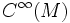$C^\infty(M)$. We then have: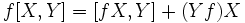$\! f[X,Y] = [fX,Y] + (Yf)X$$\! f[X,Y] = [X,fY] - (Xf)Y$

Proof

First identity

We prove this by showing that for any$g \in C^\infty(M)$, both sides evaluate to the same thing. Let's do this. Simplifying the right side yields: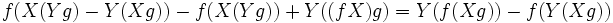$f(X(Yg) - Y(Xg)) - f(X(Yg)) + Y((fX)g) = Y(f(Xg)) - f(Y(Xg))$

Applying the Leibniz rule for$Y$ on the product of functions$f$ and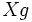$Xg$, this simplifies to:$(Yf)(Xg) + Y(Xg)(f) - f(Y(Xg)) = (Yf)(Xg)$

which is precisely equal to the left side.

Second identity

We prove this as well by taking any test function$g \in C^\infty(M)$. Simplifying the right side yields: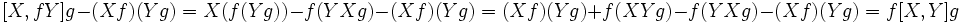$[X,fY]g - (Xf)(Yg) = X(f(Yg)) -f(YXg) - (Xf)(Yg) = (Xf)(Yg) + f(XYg) - f(YXg) - (Xf)(Yg) = f[X,Y]g$

which is the same as the left side.